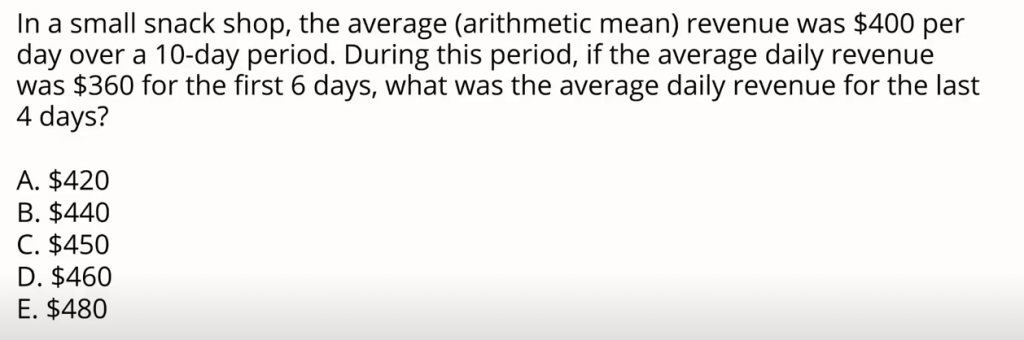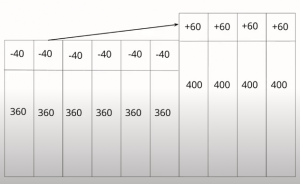The Snack shop GMAT problem is an average or a mean problem. A characteristic of many average problems is that one big takeaway right at the outset is that the answer choices are clustered tightly together. We want to refrain from making any calculations.

The problem is below:## 1. Selecting A Solution Path

If they’re looking for a level of precision, the estimation solution path isn’t available to us. However, if we dive into the problem, right from the first sentence we have sort of a conclusion that we can create via either a graphic or accounting solution path.

If you were the business owner immediately you’d say to yourself: Well for 10 days and an average of \$400 a day I made \$4000.

This is how we want to think about averages. Many times they’ll tell us a parameter about a length of time or over a certain universe of instances and here we want to treat them all as equal.

## 2. Solving the Snack Shop GMAT Problem

It doesn’t matter if one day we made 420 and another day we made 380. We can treat them in aggregate as all equal and start out with that assumption. That’s a very useful assumption to make on average problems. So, we start out knowing that we made 4,000.

What I want us to do is do a little pivot and notice from a running count standpoint how much above or below we are on a given day. So we’re told that for the first six days we averaged \$360 which means each of those six days we’re short \$40 from our average. That means in aggregate we’re short \$240 (6 days times \$40) and this has to be made up in the last 4 days.

Notice how we’re driving this problem with the story rather than with an equation. In the last four days, we need to outperform our 400 by 240. 240 divided by 4 is 60. 60 on top of the 400 target that we already have is 460. Therefore, our answer is D.

## 3. Graphical Solution PathIf we are more comfortable with graphic solution paths, imagine this in terms of 10 bars each representing \$400. Lowering six of those bars down by 40 and taking the amount that we push those first six down and distributing it among the last four bars gives us our \$460 total per day.

If you enjoyed this Snack Shop GMAT Problem, watch “The Gas Mileage GMAT Problem” next.